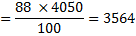## Practice Questions – Misc Aptitude – 3

1. What is 304 times 141?

(a) 39640

(b) 45942

(c) 38760

(d) 42864

(e) None of these

2. The cost of 4 rings and 2 bangles is Rs. 57200. What is the cost of 6 rings and 3 bangles?

(a) Rs. 95340

(b) Rs. 85800

(c) Rs. 76600

(d) Cannot be determined

(e) None of these

3. The difference between 54 % of a number and 26 % of the same number is 22526. What is 66 % of that number?

(a) 53097

(b) 48372

(c) 51218

(d) 49124

(e) None of these

4. Kriya deposits an amount of Rs. 65800 to obtain in a simple interest at the rate of 14 p.c.p.a. for 4 years. What total amount will Kriya get at the end of 4 years?

(a) Rs. 102648

(b) Rs. 115246

(c) Rs. 125578

(d) Rs. 110324

(e) None of these

5. The owner of a cell phone shop change his customer 28 % more than the cost price. If a customer paid Rs. 8960 for the cell phone. Then what was the cost price of the cell phone?

(a) Rs. 7800

(b) Rs. 7100

(c) Rs. 6900

(d) 6850

(e) None of these

6. In an annual examination anish scores a total of 724 marks out of 1025. What is his approximate percentage in the annual examination?

(a) 79

(b) 67

(c) 71

(d) 88

(e) 62

7. A canteen requires 651 bananas for a week Totally how many bananas will it require for the months of April. May and june 2009?

(a) 9086

(b) 8547

(c) 9284

(d) 8463

(e) None of these

8. If an amount of Rs. 436563 is distributed equally amongst 69 person, how much amount would each person get?

(a) Rs. 5943

(b) Rs. 6327

(c) Rs. 6176

(d) Rs. 5876

(e) None of these

9. There are 1825 employees in an organization, out of which 64 % got transferred to different place. How many such employees are there who got transferred?

(a) 1054

(b) 1490

(c) 1263

(d) 1168

(e) None of these

10. 64 % of a number is 2592. What is 88 % of that number?

(a)  3458

(b) 3202

(c) 3826

(d) 3564

(e) None of these

1. (d) 2. (b) 3. (a) 4. (a) 5. (e) 6. (c) 7. (d) 8. (b) 9. (d) 10. (d)

Explanations

1. 304 × 141 = 42864

2. Let the CP of 1 ring and 1 bangle be Rs. x and Rs. y respectively.

According to the question, 4x + 2y = 57200

Multiplying both sides by 1.5 we have

6x + 3y = 1.5 × 57200 = Rs. 85800

3. Let the number be x.

According to the question, (54 – 26) % of x = 22526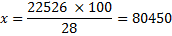66 % of 80450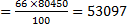4.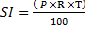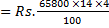= Rs.  368.48

Amount = Principal + SI

= Rs. (65800 + 36848) = Rs. 102648

5. CP of the cell phone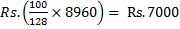6. Percentage of marks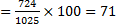7. Number of days in April, May and June 2009 = 30 + 31 + 30= 91

Requirement of bananas for 7 days = 651

Requirement for bananas for 91 days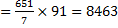8. Amount received by each person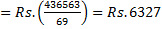9. Number of employees transferred = 64 % of 1825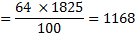10. Let the number be x.

64 % of x = 2592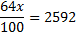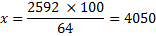88 % of 4050